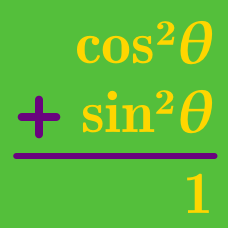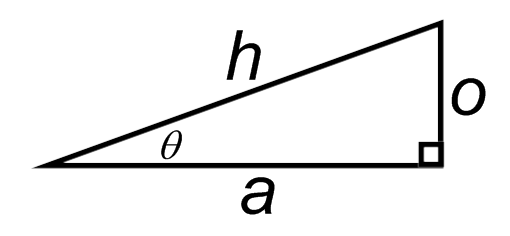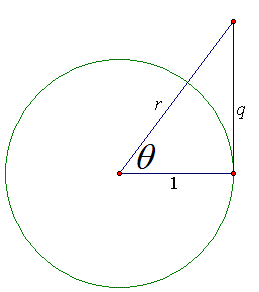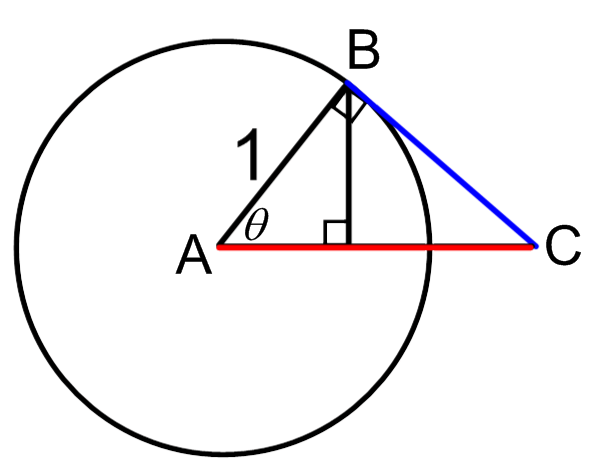Geometry

# Visualizing Pythagorean IdentitiesThe diagram above implies $a^2 + o^2 = h^2$. What Pythagorean identity is derived when both sides of the equal sign are divided by $o^2$?

Which of these equations cannot be derived from the others?Which of the following is equivalent to $r ?$Which identity is directly implied by applying the Pythagorean Theorem to triangle $ABC?$

Remember: the Pythagorean Theorem says that $AB^2 + BC^2 = AC^2.$

Which identity can be obtained by dividing both sides of $\sin^2(\theta) + \cos^2(\theta) = 1$ by $\cos^2(\theta) ?$

×